# Sort/Rank Numeric Values with Duplicate Values Exist

Excel built-in RANK function can sort a set of values. If there are duplicate numbers, then the rank number is also duplicated. See the following example: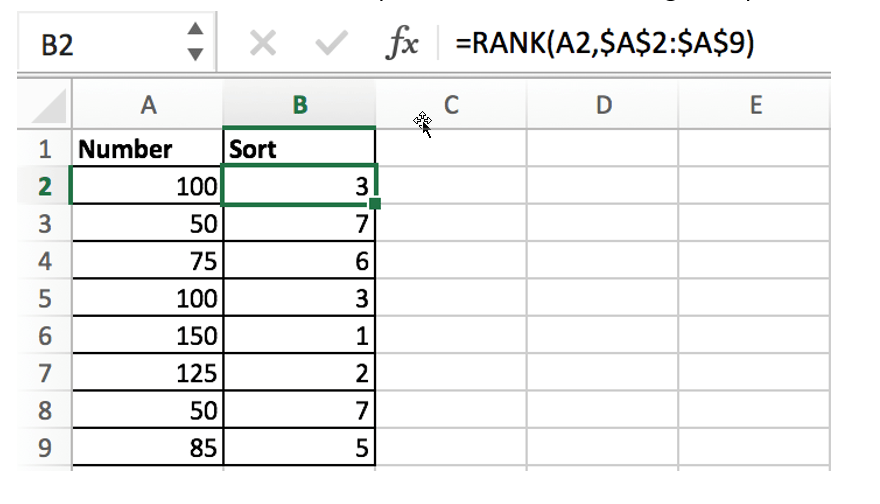There are two numbers “100” in range A2:A9, they are both the third largest number, so there are two “3” in the sort column and there is no number “4”, “4” is occupied by the second “3”.

If we want to avoid this problem (4 should be assigned to the second 100), when we encounter a duplicate number in a set of numbers, instead of assigning it the previously used rank number, we will assign it a new rank number that follows exactly the previous rank number.

See the new sequence: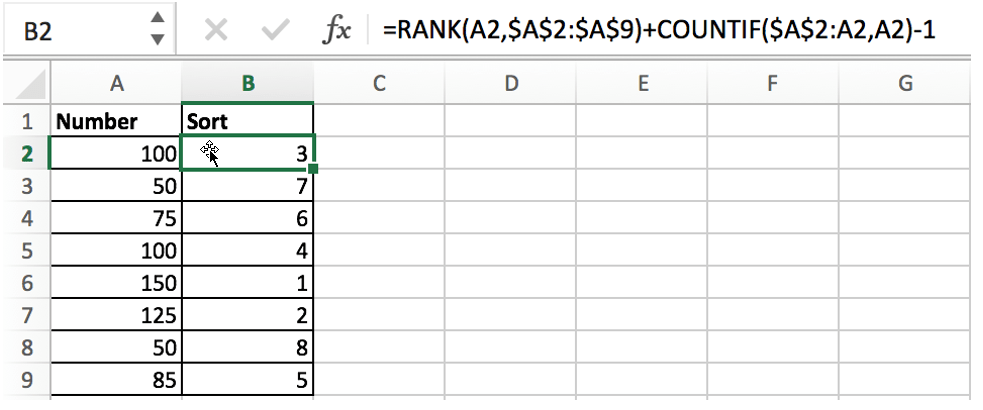## FORMULA

As we mentioned above, if you just apply the Excel RANK function to sort the numbers, once you encounter duplicate numbers, the duplicate rank number will be displayed.

When sorting numbers, we need to ensure below three points:

• Sorting numbers refer based on a proper order: ascending order or descending order
• That duplicate numbers are assigned to two numbers which are consecutive
• In Sort column, rank numbers are unique

In this example, the formula is:

`=RANK(A2,\$A\$2:\$A\$9)+COUNTIF(\$A\$2:A2,A2)-1`

Press Control+Shift+Enter to run this formula.

## EXPLANATION

We built this formula with the help of Excel RANK function and COUNTIF function, and also uses addition and subtraction operation.

### RANK EXPLANATION

The RANK function in Excel you can simply understand as rank a given set of values in ascending or descending order.

Syntax:

`=RANK(number, ref, [order])`
• number – rank this number
• ref – a set of numbers for ranking
• order – optional; ascending (1) or descending order (0)

# Ascending order means sorting the largest number as 1

# Descending order means sorting the smallest number as 1

You can set ascending or descending order according to your requirements. For example, in a race, you can set descending order when ranking time, but ascending order when ranking sales. In the example, order option is omitted, so the numbers are sorted in ascending order.

`=RANK(A2,\$A\$2:\$A\$9) – compare the number in cell A2 with the numbers in the range \$A\$2:\$A\$9`

In this example, the numbers are arranged in a fixed range. To avoid the range being adjusted when copying the formula, so we add \$ to lock the range. This formula works well if there are no duplicate numbers, and the RANK function will assign 1 to the largest number and 2 to the second largest number according to the rule, and so on.

Unfortunately, there are duplicate numbers in this example. To break the tie caused by the limitations of the RANK function, we added COUNTIF function to enhance the formula.

### COUNTIF EXPLANATION

Excel COUNTIF function returns the number of cells the meet one condition.

Syntax:

`=COUNTIF(range, criteria)`

In this example, the formula is:

`=COUNTIF(\$A\$2:A2,A2) – returns how many duplicate numbers in the selected range`

When copying the formula downward, the given range \$A\$2:A2 is extended, the starting cell A2 is locked, and the ending cell is the cell where the RANK function is currently working. Therefore, if we encounter a duplicate number in the range, the COUNTIF function will return the number of times the number is duplicated.

• If number only appears once, COUNTIF returns 1 as current row is included in selected range;
• If number appears twice, COUNTIF returns 2, and so on;
`=COUNTIF(\$A\$2:A2,A2)-1`

Since the number appears at least once in the selected range, for example, COUNTIF(\$A\$2:A2,A2) returns 1, we use the subtraction operation “-1” to offset the value returned by COUNTIF.

### FORMULA EXPLANATION

We take number “100” in cell A2 as an example, in B2 enter below formula:

`=RANK(A2,\$A\$2:\$A\$9)+COUNTIF(\$A\$2:A2,A2)-1`

Convert cell or range reference to actual number or array, the formula is:

`=RANK(100,{100;50;75;100;150;125;50;85})+COUNTIF(100,100)-1`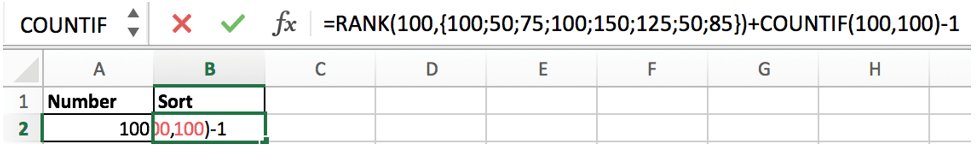As number “100” is the third largest number in the array, so “3” is returned by RANK function; COUNTIF function returns “1”, so the result is “3+1-1=3”.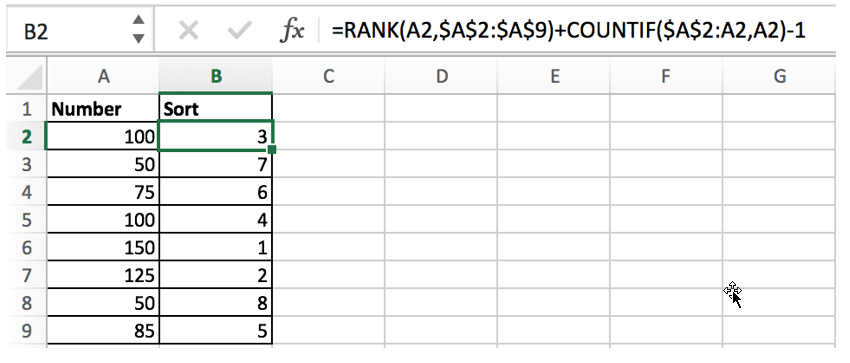We can see that the formula returns “4” for the same number “100” located in cell A5. This time COUNTIF function plays an important role and breaks tie perfectly.

In B5, the formula is:

`=RANK(A5,\$A\$2:\$A\$9)+COUNTIF(\$A\$2:A5,A5)-1`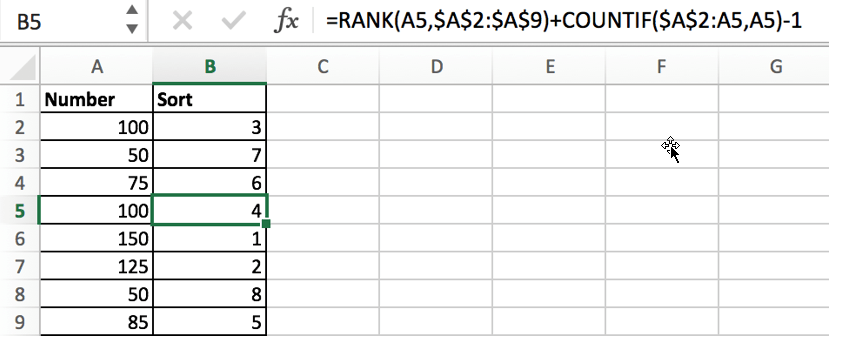RANK returns 3:

`-> 3+COUNTIF({100;50;75;100},100)-1`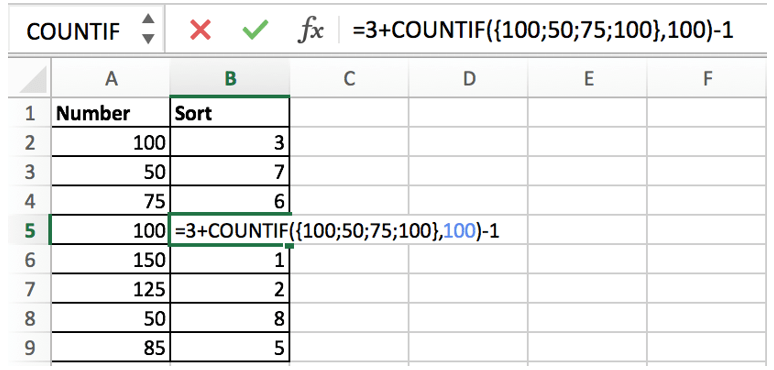COUNTIF returns 2 because {100;50;75;100} contains two “100”:

`-> 3+2-1 -> 4`

### Related Functions

• Excel COUNTIF function
The Excel COUNTIF function will count the number of cells in a range that meet a given criteria. This function can be used to count the different kinds of cells with number, date, text values, blank, non-blanks, or containing specific characters.etc.= COUNTIF (range, criteria)…
• Excel Rank function
The Excel RANK function returns the rank of a given number in a supplied range of cells.  If there are duplicated values in a list of values, it will be set the same rank.The syntax of the RANK function is as below:= RANK (number,ref,[order])….
Related Posts

Calculate Days Open in Excel

If you want to know how to Calculate days in Excel, there are some formulas that you can use to do so. For example, you can use the DAYS function in Excel to find the number of days between two ...

Calculate Grades With VLookup in Excel

Why Should You Calculate Grades With VLookup Excel? If you're looking for a simple way to find out a student's grades, VLOOKUP Excel can do the trick. This function uses a lookup table to find the values and sort them ...

Repeating Character n Times in Excel

In daily life, we can use repeated characters to indicate the magnitude or priority of something. For example, a single “!” indicates a minor case, double “!!” indicates a medium case, and triple “!!!” indicates a high case, and so ...

Calculate Cumulative Loan Interest in Excel

What is Cumulative Loan Interest? When comparing different types of loans, many people want to know what is Cumulative Loan Interest. The sum of all interest payments you've made on a loan is referred to as cumulative interest. Different lenders ...

Calculate Cumulative Loan Principal Payments in Excel

How do you Calculate Cumulative Loan Principal Payments in Excel? There are a few different ways to calculate this information, but one way has been proven to be accurate more often than others. One option is to use the CUMPRINC ...

Trap Error or Replace Error by Specific Value with IFERROR function

We often use Excel formulas in our work life, and we may encounter this situation that the formula throws an error and finally an error like #DIV/0! Is displayed in the cell. In today’s tutorial, we will introduce you the ...

Calculate Compound Interest in Excel

This article will show you how to calculate compound interest in Excel. It will help you calculate the interest you will accrue on a given amount of money. There are several ways to calculate compound interest. One of the simplest ...

Count Attendance and Absence with COUNTIF function

In our campus life and work life, we usually record everyone's attendance. Today we will introduce you the application of Excel COUNTIF function to count the attendance. Using a week as an example, we use the following example to show ...

Find the Closest Data to the Data Provided in Excel

In our daily work, we may encounter such an issue that to find the closest value to a certain value. In fact, Excel internal functions can help us solve this problem. In today’s article, we will show you how to ...

Average Of Numbers With Multiple Criteria In Excel

Have you ever come across a task to calculate the average of the numbers with respect to multiple criteria? Are you tired of doing this cumbersome task manually? Are you willing to do this task smartly in just a matter ...

Sidebar